International
Tables for
Crystallography
Volume B
Reciprocal space
Edited by U. Shmueli

International Tables for Crystallography (2006). Vol. B. ch. 2.1, p. 205   | 1 | 2 |

## Section 2.1.8.3. Simple examples

U. Shmuelia* and A. J. C. Wilsonb

aSchool of Chemistry, Tel Aviv University, Tel Aviv 69 978, Israel, and bSt John's College, Cambridge, England
Correspondence e-mail:  ushmueli@post.tau.ac.il

#### 2.1.8.3. Simple examples

| top | pdf |

Consider the Fourier coefficient of the p.d.f. offor the centrosymmetric space group. The normalized structure factor is given by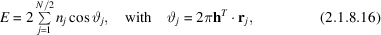and the Fourier coefficient is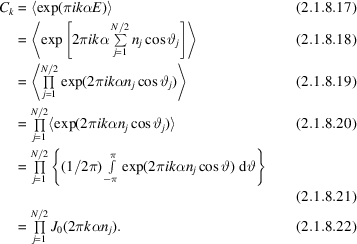Equation (2.1.8.20)is obtained from equation (2.1.8.19)if we make use of the assumption of independence, the assumption of uniformity allows us to rewrite equation (2.1.8.20)as (2.1.8.21), and the expression in the braces in the latter equation is just a definition of the Bessel function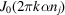(e.g. Abramowitz & Stegun, 1972).

Let us now consider the Fourier coefficient of the p.d.f. offor the noncentrosymmetric space group. We have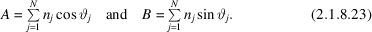These expressions for A and B are substituted in equation (2.1.8.10), resulting in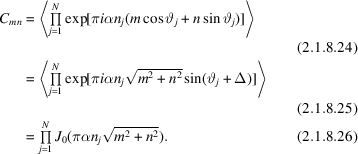Equation (2.1.8.24)leads to (2.1.8.25)by introducing polar coordinates analogous to those leading to equation (2.1.8.8), and equation (2.1.8.26)is then obtained by making use of the assumptions of independence and uniformity in an analogous manner to that detailed in equations (2.1.8.12)–(2.1.8.22)above.

The right-hand side of equation (2.1.8.26)is to be used as a Fourier coefficient of the double Fourier series given by (2.1.8.9). Since, however, this coefficient depends on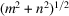alone rather than on m and n separately, the p.d.f. offor P1 can also be represented by a Fourier–Bessel series [cf. equation (2.1.8.11)] with coefficient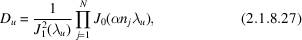where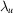is the uth root of the equation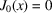.

### ReferencesAbramowitz, M. & Stegun, I. A. (1972). Handbook of mathematical functions. New York: Dover.Google Scholar